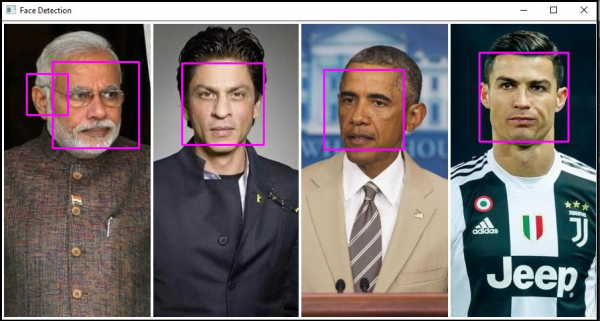# How to detect the face in still picture in OpenCV using C++?

OpenCVC++Server Side ProgrammingProgramming

We detect the faces from the image. To detect the face, we used 'detectMultiScale()' function.

The actual format of this function is −

## Syntax

detectMultiScale(source matrix, vector, searchScaleFactor, minNeighbours, flags, minfeatureSize)

By changing the function arguments, we can control the 'detect.MultiSpace()' function. This function takes the following arguments.

### Source Matrix

It is the matrix where the face will be detected. In this case, it will the matrix which is keeping the video frames.

### Vector

The 'detect.MultiScale()' function will be a vector of rectangular type. A rectangle is a vector in OpenCV, and we have to define it as a vector.

### searchScaleFactor

Search scale factor determines how many different sizes of faces the function will look for. We usually use 1.1. If necessary, we can use 1.2 to make the detection system faster. However, in this case, the faces are not detected as frequently as when 1.1 is used.

### minNeighbours

This parameter detects the confidence level of the detector. It means this function shows how confident the detector is that it has detected a face. For better reliability, we can use a higher number, but it will slow down the process. For a faster process but lower reliability, we can use the smaller number. We usually use 3 or 4 as minNeighbours.

### flags

By default, the function will look for all the faces. If we use 'CASCADE_FIND_BIGGEST_OBJECT' as the flag's value, it will only look for the largest face. In this case, the system performs faster. Using 'CASCADE_SCALE_IMAGE', we can search for multiple faces.

### minFeatureSize

minFeatureSize determines the minimum size of the face. If we want to detect faces located far away from the camera, then we should use smaller value. If the faces are close to camera, we should use larger value. We use (20 x 20) or (30 x 30) size for typical distance. In the example, we used detectMultiScale() function as

faceDetector.detectMultiScale(image_with_humanface, faces, 1.1, 4, CASCADE_SCALE_IMAGE, Size(20, 20));//

The following code demonstrates how to detects human faces from a still picture in OpenCV.

## Example

#include<iostream>
#include<opencv2/highgui/highgui.hpp>
#include<opencv2/imgproc/imgproc.hpp>
//This header includes definition of 'rectangle()' function//
#include<opencv2/objdetect/objdetect.hpp>
#include<string>
using namespace std;
using namespace cv;
int main(int argc, char** argv) {
Mat image_with_humanface;//Declaring a matrix to load image with human faces//
namedWindow("Face Detection");//Declaring a window to show the result//
string trained_classifier_location = "C:/opencv/sources/data/haarcascades/haarcascade_frontalface_alt.xml";//Defining the location our XML Trained Classifier in a string//
vector<Rect>faces;//Declaring a rectangular vector named faces//
vector<Rect>boundary;//Declaring a rectangular vector named rectangle//
faceDetector.detectMultiScale(image_with_humanface, faces, 1.1, 4, CASCADE_SCALE_IMAGE, Size(20, 20));//Detecting the faces in 'image_with_humanfaces' matrix//
for (size_t i = 0; i < faces.size(); i++){ //Loop to draw rectangle around the faces//
Mat faceROI = image_with_humanface(faces[i]);//Storing the face in a matrix//
int x = faces[i].x;//Getting the initial row value of face rectangle's starting point//
int y = faces[i].y;//Getting the initial column value of face rectangle's starting point//
int h = y + faces[i].height;//Calculating the height of the rectangle//
int w = x + faces[i].width;//Calculating the width of the rectangle//
rectangle(image_with_humanface, Point(x, y), Point(w, h), Scalar(255, 0, 255), 2, 8, 0);//Drawing a rectangle using around the faces//
}
imshow("Face Detection", image_with_humanface);//Showing the detected face//
waitKey(0);//To wait for keystroke to terminate the program//
return 0;
}

## Output